ISIJ International
Online ISSN : 1347-5460
Print ISSN : 0915-1559
ISSN-L : 0915-1559
Forming Processing and Thermomechanical Treatment
A New Curvature Analytical Method in Plate Leveling Process
Zhifang LiuYuanxin LuoYongqin WangThunshun Warren Liao
Author information
JOURNALS OPEN ACCESS FULL-TEXT HTML

2018 Volume 58 Issue 6 Pages 1094-1101

Details
Abstract

Leveling is a process used to minimize the plate defects including flatness imperfections and uniformity of internal stress in the plate industry. It plays an important role in delivering the desired material properties and product standards required. This paper presents a new analytical method for the curvature analysis of plate during the leveling process. The explicit expressions of the curvature integration model were derived in detail by an assumption of ignoring the residuals stress superimposed effect (IRSSE). The curvature analysis procedure was given about how to choose different expression to build the explicit curvature integration equations which were solved with fsolve function in Matlab. The contact angles and bending curvatures could be firstly analyzed in 0.7–0.8 second even though with random initial values. Then, they were initialized to the procedure by considering the residual stress effect and it only needed 3–4 seconds in 2 iteration steps to get the final solution. It was found that the result by IRSSE is close enough to the solution of the method by considering residual stress effect (CRSSE). The curvature and trajectory distribution by IRSSE and CRSSE just reflects on the last leveling areas because of the residual stress accumulative error effect. The analytical methods by IRSSE and CRSSE have the advantage of iteration speed and solution accuracy, respectively. Therefore, the proposed combined method is potential to the future roller intermeshes optimization research.

1. Introduction

A variety of standard sizes of plate are usually used for general engineering purposes. However, conventional plate rolling process, cooling transformation, improper placement during transport and non-uniformity distribution of residual in-plane normal stress along the thickness direction often yields some defects including curl, gutter, middle waves and edge waves.1) To ensure effective correction of the aforementioned defects and quality improvement in the production of plates, the leveling process must be properly designed to reduce these defects before it goes to the secondary manufacturing process.

Bending process is a simple reverse application of the leveling process. Therefore, it is easy to get the explicit expressions of the deflection and force of the plate.2,3,4) However, there are several times of alternating bending and reverse bending operations in the leveling process. Therefore, it is difficult to derive the explicit expressions of the curvature and trajectory for the plate in the leveling process. Based on the literature survey, numerous research studies have been performed to model and predict the leveling process by taking different approaches. Several researchers simulated and predicted the leveling process by using the finite element method (FEM).5,6,7,8,9,10) Although the FEM result have good consistence with the experimental result, it is difficult to use this method in practice because it is time-consuming.

Dratz et al.11) proposed a finite element approach, an analytical and a semi-analytical model to determine the loading modes experienced by the material in roll leveling operations. Their findings include that the semi-analytical model is the more appropriate in order to determine the parameters of rolls levelers and the analytic one is sufficient to define the size of the levelers. Fischer et al.12) carried out analytical and numerical studies of buckling phenomena in thin plates or strips, proposed an effective model for eliminating residual stress, and developed mathematical models of estimating wave shape of buckling. Yin et al.13) studied the section steel leveling process on a flexible roller system based on spring back theory. They found that the flexibility of the last rollers is helpful to improve the leveling quality. Morris et al.14) studied the effect of leveling process parameters on the shape characteristics of the leveled product. In order to investigate the essential deformation law of leveling for plates with transverse waves, Chen et al.15) proposed a 2.5-dimensional analytical approach. They transversely divided the plate into some strips with equal width in the approach. They found that the bending of roller is a main approach to eliminate the transverse waves. Ye et al.16) developed an on-line model predicting the residual stress in roller leveling process by assuming the plain strain condition and ignoring the stress in thickness direction. To validate the accuracy of the model, the camber and gutter deformation of the real sized plate were measured and compared with the model predicted values. Moreover, the variations of residual stress were studied according to the entry and the delivery intermeshes, respectively. To simulate the leveling process, Doege et al.17) developed an analytic forming model to analyze the leveling process in a shorter time than that by using the FEM at the Institute of Hanover University. Kadota et al.18) and Higo et al.19) improved the originally proposed the curvature integration model which describes the relationship between the curvature and reduction of rollers. They solved it by using an algorithm with eight steps and two loops. However, it may be easy to fail to achieve the final results if any loop is not convergent. Xue et al.20,21) used a decoupled method to solve Kadota and Higo’s model for obtaining curvature distribution. The convergence of simulation results still depends largely on contact angles. Lee et al.22) proposed a relative simple and effective method to design the leveling process with three rollers for the molten carbonate fuel cell collector. Their method can calculate the optimal bending curvature and roll intermeshes of the middle roller to flat the collector.

To further accelerate the analytical speed of the curvature integration model, this paper presents a new method to solve the curvature integration model by the combination of both ignoring and considering the residual stress superimposed effect. The rest of this paper is organized as follows. The curvature integration model is introduced again and the explicit expressions of curvature integration are derived in detail in Section 2. In Section 3, the curvature analytical speed and solution accuracy are compared between the proposed analytical method and the method in reference . Finally, the conclusions are given in Section 4.

2. The Proposed Curvature Analytical Method

The basic curvature integration model in reference  can be summarized as follow

 θ x = ∫ 0 x κ x dx ± θ i-1 (1)

 z x = ∫ 0 x ∫ 0 x κ x dxdx ± θ i-1 x (2)
where the sign in Eqs. (1) and (2) depends on the roller ID., i. All rollers are numbered from left to right with the leftmost bottom roller numbered as 1, as shown in Fig. 1. There are two relative positions between the plate and rollers in Fig. 1. If i can be divided exactly by 2, the sign in Eq. (1) is ‘+’ while it is ‘−’ in Eq. (2). Otherwise, the sign in Eq. (1) is ‘−’ while it is ‘+’ in Eq. (2). θx, zx and κx are the angle, vertical ordinate and bending curvature of point B, respectively, θi−1 is the contact angle between the plate and roller i−1.Fig. 1.

Schematic layout of the curvature integration model.

When point B moves to point C in Fig. 1, Eqs. (1) and (2) can be further derived as

 θ x | x= L i-1 ± θ i =0 (3)

 ∓ z x | x= L i-1 +R( 1-cos θ i-1 ) +R( 1-cos θ i ) = δ i (4)
where θi is the contact angle between the plate and roller i, Li−1 is the distance between contact point A and C, L i-1 = p 2 +Rsin θ i-1 -Rsin θ i , δi is the downfeed of upper roller ialong the z axis. p and R denote the inter roller distance and radius of each roller (all rollers are of the same size), respectively. Roller 1 and 9 are usually needed to be moved upward or downward slightly for roll-bite and further leveling quality improvement purpose. It is necessary to leave room to install driving devices. Therefore, p1 (distance between roller 1 and 2) and p8 (distance between roller 8 and 9) are larger than p 2 .

The curvature integration model was successfully solved by building nonlinear implicit equations with residual stress superimposed effect consideration in reference . But it needs about 15 seconds and 7 times iterations to get the solution. To apply the curvature integration model to the roller intermeshes optimization research, it is necessary to find a faster analytical method.

Many materials models have been proposed for the mild steels, including elastic perfectly plastic models, elastic linear strain hardening models, elastic power-law strain hardening models and pure power-law strain hardening models. The elastic perfectly plastic model is firstly used to capture the characteristic of material to deduce the curvature integration explicit expression. The bending stress in the outer layers of the plate begin to be forced into elastic state when the cross section a-a’ in Fig. 2 moves from point O to A. The outer layer reaches the maximum elastic-plastic state for the first time when the section a-a’ moves to point Blocated at the contact point between the plate and roller 2. Subsequently, section a-a’ start to release until the inner stress is self-balanced at point D, as shown in Fig. 2(d). Note that layers forced to get elastic-plastic deformation cannot recover to their initial state in the releasing process and there must exist residual stress distribution and residual curvature, 1/ρc1. The residual stress distribution and residual curvature are the initial stress and curvature of the next bending process, respectively, as shown from Figs. 2(d) to 2(e). Then, the layers with residual stresses begin to be bent in the inverse direction from point E to F. This process continues until the plate passes through the whole leveling area.24)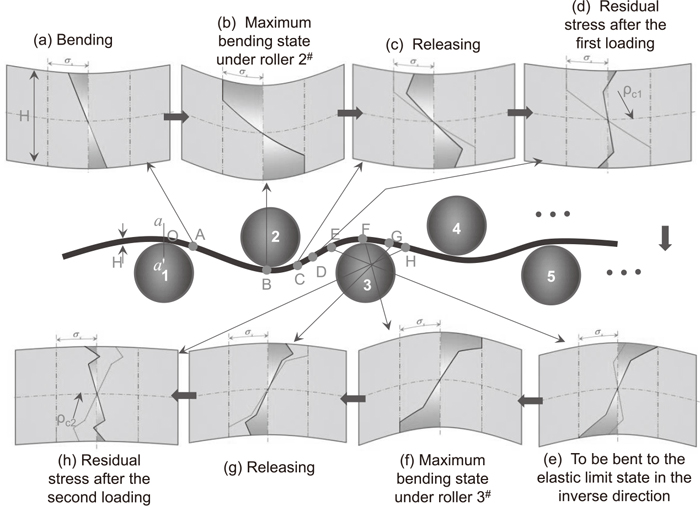Fig. 2.

True stress distribution during leveling process.

Here, a tentative assumption is made as follow: by ignoring the residual stress superimposed effect but retaining the residual curvature superimposed effect, as shown from Figs. 3(d) to 3(h). Taking the residual stress state of a section located at point D as an example, the residual stress is reset to 0 while it is actually distributed in ‘X’ shape, as shown in Fig. 2(d). It means that the initial stresses in the thickness direction after each bending process are reset to zero distribution. The advantage of this assumption is that the bending stress in each leveling area starts from zero distribution and there is no need to consider the ‘X’ shape initial stress for the next bending process. Hence, it is possible to express explicitly the inner moment of the plate under each plate.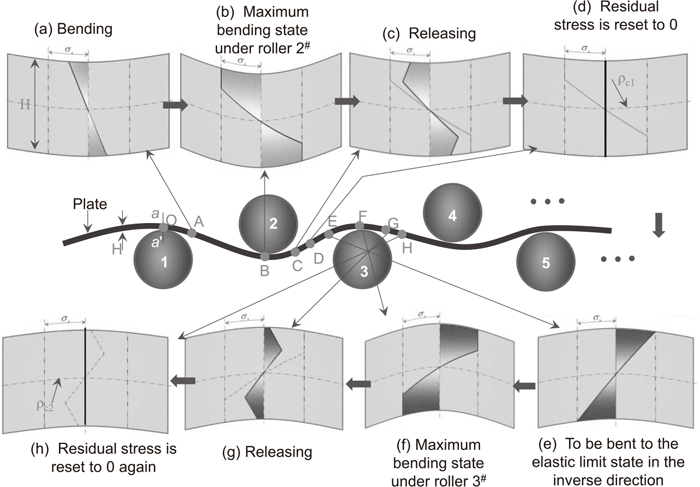Fig. 3.

The inner bending moment of the plate under roller i, Mi, can be expressed as

 { M ei = ∫ - B 2 B 2 ∫ - H 2 H 2 σ(x)zdydz M pi = ∫ - B 2 B 2 ∫ - H t 2 H t 2 σ(x)zdydz +2 ∫ - B 2 B 2 ∫ H t 2 H 2 σ s zdydz (5)
where Mei is the inner elastic bending moment if the outer layer is only forced into the elastic state, Mpi is the inner elastic-plastic bending moment if the outer layer is forced into the elastic-plastic state, B and H are the width and thickness of the plate, respectively, Ht is the half thickness of elastic layers, σs is the yield stress of the plate, σ(x) is the bending stress, as shown in Fig. 4.Fig. 4.

Stress state in the thickness direction.

Considering the leveling process as a unidirectional bending process without tensile forces, the bending stress, σ(x), is proportional to the bending strain, ε(x), with the slope of Young modulus of the plate, E,

 σ(x)=Eε(x)=E( κ i - κ 0i )z (6)
where κi is the bending curvature of the plate under roller i, κ0i is the initial curvature the plate for the next bending process.

Substituting Eq. (6) into Eq. (5), the inner bending moment can be expressed as

 { M ei =EI( κ i - κ 0i )< M t                                      (a)   M pi = M t ( 1.5- 0.5 C Σ 2 ) ≥ M t                          (b) (7)
where I is the inertia moment of the geometric section, I= B H 3 12 , C = κ κ t = κ i - κ 0i κ t , Mt is the elastic limit bending moment, M t = BH σ s 2 6 , κt is the elastic limit curvature, κ t = 2 σ s EH .

Taking the case of Fig. 1(A) as an example, there is no external force between adjacent rollers. Therefore, the moment Mx between roller i−1 and i can be linearly expressed as

 M x =- M i-1 + M i-1 + M i L i-1 x (8)
where Mi−1 and Mi are the inner bending moment of the plate located at roller i−1 and roller i, respectively.

The curvature of point B, κex, in Fig. 1(A), if there is no elastic-plastic deformation for the plate under roller i shown in Fig. 5, can be expressed as

 κ ex = κ 0i + M x EI (9)
where κex is the curvature distribution in the elastic deformation part.Fig. 5.

Moment and curvature distribution of elastic deformation state.

Substituting Eq. (8) into Eq. (9),

 κ ex = κ 0i - M i-1 EI + M i-1 + M i EI L i-1 x (10)
Substituting Eq. (10) into Eqs. (1) and (2),
 θ ex =( κ 0i - M i EI ) x+ M i-1 + M i 2EI L i-1 x 2 + θ i-1 (11)

 z ex = 1 2 ( κ 0i - M i-1 EI ) x 2 + ( M i-1 + M i ) 6EI L i-1 x 3 + θ i-1 x (12)
where θex and zex are the explicit expressions of angle and coordinate at the elastic deformation area in Fig. 1(A), respectively.

The bending stress releases completely at point B in Fig. 5 because the inner bending moment decreases to zero. The residual curvature and stress recover to their initial state because there is no elastic-plastic deformation for the plate under roller i. However, the situation is different if the plate is forced into elastic-plastic deformation under roller i, as shown in Fig. 6. The plate begins to release after it leaves roller i−1 until its inner moment reduces to zero. Then, it starts to be bent reversely into the elastic state until the inner moment arrives at the elastic limit bending moment, Mt. Finally, the outer layer reveals yield state and the curvature changes nonlinearly from point B to C.Fig. 6.

Moment and curvature distribution.

The curvature explicit expression from point O to B in Fig. 6 is the same with Eq. (10). But it is much more complex from point B to C because of the nonlinear characteristic of the material. According to Eq. (7)-(b), the bending curvature can be deduced as,

 κ px = κ 0i - κ t M t 3 M t +2 M x (13)
where κpx is the curvature distribution in the elastic-plastic deformation area (from point B to C in Fig. 6).

Substituting Eq. (8) into Eq. (13),

 κ px = κ 0i - κ t M t L i-1 (3 M t +2 M i-1 ) L i-1 -2( M i-1 + M i )x (14)

Substituting Eq. (14) into Eqs. (1) and (2), the angle and coordinate of point B at the plastic deformation area in Fig. 1(A) can be derived as

 θ px = ∫ L t i x κ px dx + θ ex | x=L t i                = ∫ M t + M i-1 M i-1 + M i L i-1 x [ κ 0i - κ t M t L i (3 M t +2 M i-1 ) L i -2( M i-1 + M i )x ]                          dx+ θ tx | x= M t + M i-1 M i-1 + M i L i-1                = κ 0i x- M t M t L i-1 EI( M i + M i ) (3 M t +2 M i-1 ) L i-1 -2( M i-1 + M i )x                + (3 M t 2 - M i-1 2 ) 2EI( M i-1 + M i ) L i-1 + θ i-1 (15)

 z px = ∫ L t i x θ px dx + z ex | x=L t i = ∫ M t + M i-1 M i-1 + M i L i-1 x θ sx dx+ z ex | x= M t + M i-1 M i-1 + M i L i-1                 = 1 2 κ 0i x 2 + A t M t L i-1 3 ( M i-1 + M i ) 2 [ (3 M t +2 M i-1 ) L i-1 -2( M i-1 + M i )x ] 3 2                   +[ (3 M t 2 - M i-1 2 ) L i-1 2EI( M i-1 + M i ) + θ i ]x- (10 M t 3 - M i-1 3 +9 M i-1 M t 2 ) L i-1 2 6EI ( M i-1 + M i ) 2 (16)
where Lti is the distance from point O to B as shown in Fig. 6, L t i = M t + M i-1 M i + M i L i-1 , θpx and zpx are the angle and coordinate function at the elastic-plastic deformation area, respectively.

The relative position between the plate and rollers is different in Figs. 1(A) and 1(B). The angle and coordinate explicit expression of the case in Fig. 1(B) are much similar with the Eqs. (11), (12) and (15), (16) expect some signs which can be expressed as

 θ ex =( κ 0i + M i EI ) x- M i-1 + M i 2EI L i-1 x 2 - θ i-1 (17)

 z ex = 1 2 ( κ 0i + M i-1 EI ) x 2 - ( M i-1 + M i ) 6EI L i-1 x 3 - θ i-1 x (18)

 θ px = ∫ L t i x κ px dx + θ ex | x=L t i                = ∫ M t + M i-1 M i-1 + M i L i-1 x [ κ 0i + κ t M t L i (3 M t +2 M i-1 ) L i -2( M i-1 + M i )x ]                          dx+ θ tx | x= M t + M i-1 M i-1 + M i L i-1                = κ 0i x+ M t M t L i-1 EI( M i + M i ) (3 M t +2 M i-1 ) L i-1 -2( M i-1 + M i )x                  -      (3 M t 2 - M i-1 2 ) 2EI( M i-1 + M i ) L i-1 - θ i-1 (19)

 z px = ∫ L t i x θ px dx + z ex | x=L t i = ∫ M t + M i-1 M i-1 + M i L i-1 x θ sx dx+ z ex | x= M t + M i-1 M i-1 + M i L i-1 = 1 2 κ 0i x 2 + A t M t L i-1 3 ( M i-1 + M i ) 2 [(3 M t +2 M i-1 ) L i-1 -2( M i-1 + M i )x] 3 2         + [ (3 M t 2 - M i-1 2 ) L i-1 2EI( M i-1 + M i ) + θ i ]x- (10 M t 3 - M i-1 3 +9 M i-1 M t 2 ) L i-1 2 6EI ( M i-1 + M i ) 2 (20)

Substituting Eqs. (11), (12), (15), (16) and (17), (18), (19), (20) into Eqs. (3), (4), the explicit curvature integration expression can be built. The procedure of building explicit curvature integration equations by ignoring the residual stress superimposed effect is shown in Fig. 7. First, the bending curvatures from roller 2 to N−1 and contact angles from roller 1 to Nare randomly initialized, as well as a matrix λ consisted of 2×(N−1) zeros. N is the total number of rollers. Then, the elastic-plastic stress state of the leveled plate is easy to be determined according to the comparison between the inner bending moment and the elastic limit bending moment in Eq. (7). Next, the curvature integration explicit equation expression is chosen according to the number of the leveling area and the elastic-plastic stress state of the plate under roller i. Thirdly, the residual curvature is calculated according to the residual stress self-balanced principle. Note that the residual stress should be set to 0 in this step so that the explicit expression can be derived. This process continues until the cross section a-a’ in Fig. 2 exits the leveling area. Finally, the matrix consisted of 2×(N−1) equations is assembled and solved. All programs are carried out by the fsolve function in Matlab.Fig. 7.

Procedure of building explicit curvature integration equations by IRSSE.

3. Verification and Discussion

The procedure about how to build and solve the nonlinear implicit curvature integration equations was proposed in detail in literature , as well as experimental verification study. Therefore, their data can be used to make a comparative study in this paper. The initial parameters are also employed as list in Table 1.

Table 1. Leveling conditions employed in the proposed method.23)
Number of work rollersN9 (top: 4, bottom: 5)
Roller diameter (mm)D280
Inter-roller distance (mm)p300
Distance between roller 1 and 2 (mm)p1190
Distance between roller 8 and 9 (mm)p8190
Yield stress (MPa)σs127.6
Young’s Modulus (MPa)Ε115900
Plate thickness (mm)H20
Plate width (mm)B3000
Entrance inter-mesh (mm)δ21.32
Exit inter-mesh (mm)δ80

To find out the convergence of the proposed analytical method, the curvatures, κi (i=2–8), and contact angles, θi (i=1–9), in Fig. 7 are randomly initialized three times from −2 to 6 degrees and 0 to 0.6/m, respectively, as shown in Figs. 8 and 9. It only needs about 0.7–0.8 second to get the desired solution in seven iteration steps. Actually, the searched value in the fourth step is close enough to the final solution. The bending curvatures of roller 1 and roller 9 are the initial and residual curvature, respectively. Therefore, Fig. 9 only shows the absolute values of the bending curvatures from roller 2 to 8.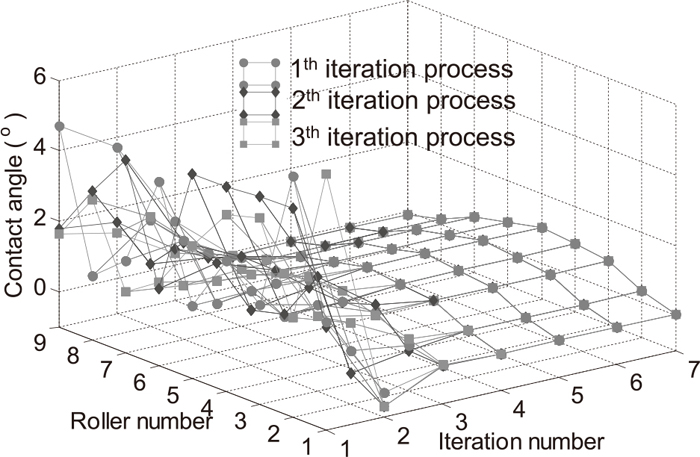Fig. 8.

Contact angle iteration process by IRSSE.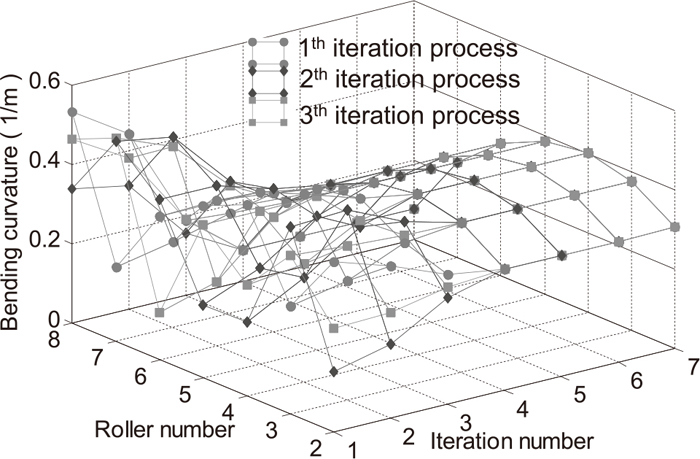Fig. 9.

Absolute values of bending curvature iteration process by IRSSE.

The solution found in Figs. 8 and 9 does not consider the residual stress superimposed effect. To make a comparative study, the contact angles and bending curvatures loaded from Figs. 8 and 9 are firstly taken as the initial value. Then, they are initialized to the procedure in reference . The contact angle and curvature distributions which consider the residual stress superimposed effect are depicted in Figs. 10 and 11, respectively. It only needs 3–4 seconds to get the actual curvature distribution. It can be found that the solution in the second iteration completely coincide with the analytical result in reference  which needs 7 iteration steps in 15 seconds. The reason is that the analytical method in reference  needs to slice the plate into numbers of elements in the thickness and length direction for discretization purpose because of the complex stress distribution in the thickness direction.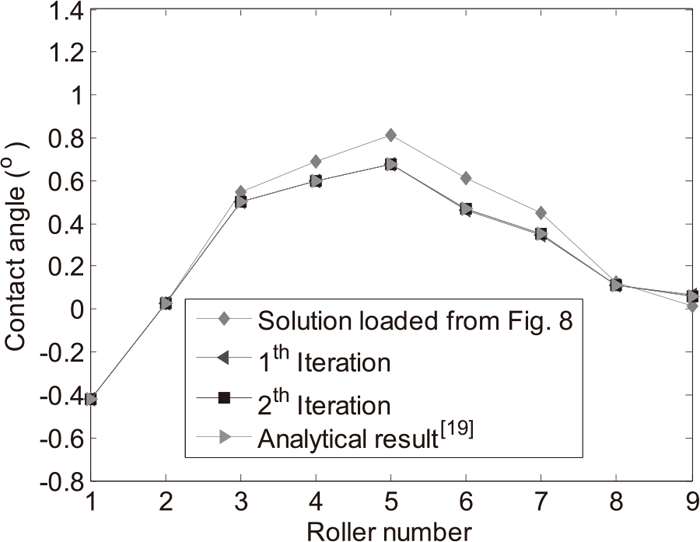Fig. 10.Fig. 11.

To further investigate the influence of the ignoring the residual stress superimposed effect on the curvature analysis, the factors of initial curvature and hardening coefficient are considered. It can be found that the curvature distributions during the leveling process are close enough except the last leveling area, as shown in the part A of Figs. 12 and 13. It means that the accumulative error effect of the residual stress reflects in the last leveling areas.Fig. 12.

Curvature distribution during the leveling process by IRSSE.Fig. 13.

Curvature distribution during the leveling process by CRSSE.

The corresponding trajectory for different initial curvatures can also be calculated in Figs. 14 and 15. The most obvious difference still locates at the last leveling region because they are integrated twice from Figs. 12 and 13, respectively.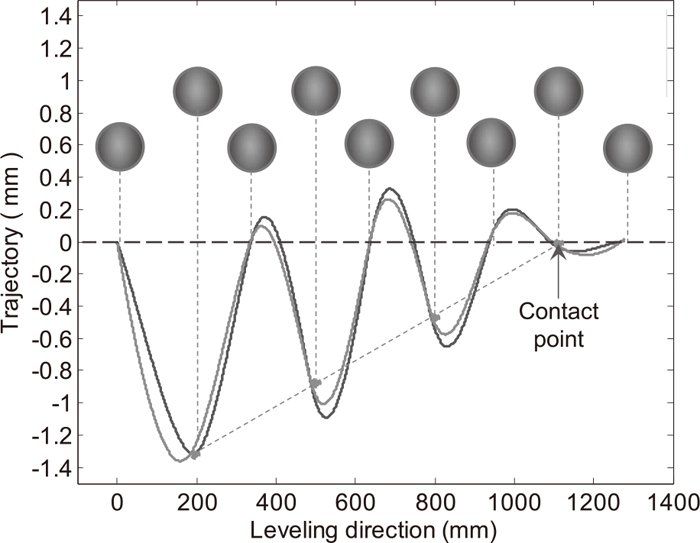Fig. 14.

Trajectory distribution during the leveling process by IRSSE.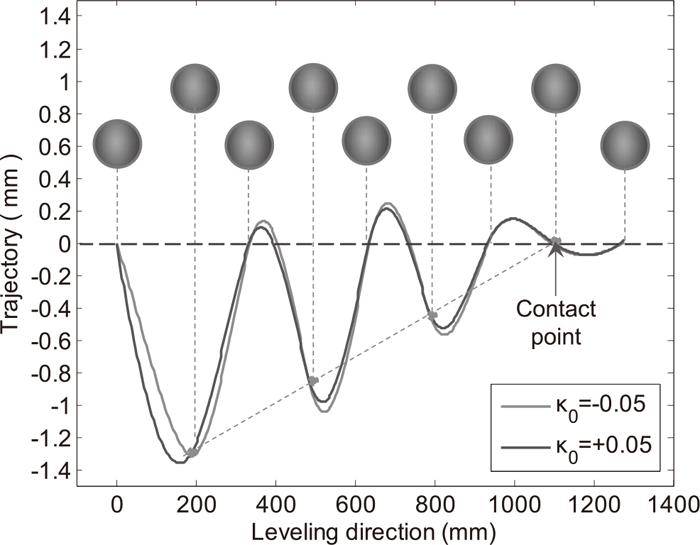Fig. 15.

Trajectory distribution during the leveling process by CRSSE.

The influence of the hardening coefficient on the curvature distribution is shown in Fig. 16. It can be seen that the differences still locate at the exit leveling areas. The reason is that the hardening effect becomes increasingly obvious with the more elastic-plastic deformation times.Fig. 16.

Curvature analysis comparison for different hardening coefficient.

The most obvious advantage of the proposed analytical method is to derive the curvature integration equations explicitly. They can be used directly to analyze the curvature distribution during the leveling process. There is no need to consider the elastic-plastic deformation state of the plate between adjacent rollers in the curvature analytical process. The reason is that it is unnecessary to cut the plate into sets of elements like the method in reference  because the expressions of the angle and coordinate are explicit. Therefore, the proposed combined analytical method not only satisfies the solution accuracy but also accelerates the solution speed.

4. Conclusions

This paper presents a new method for the curvature analysis of the plate leveling process. Based on the result presented above, it is believed that the following conclusions can be drawn:

(1) The curvature integration model can be explicitly expressed by ignoring the residual stress superimposed effect during the elastic-plastic and continuous alternating bending process. The selection of angle and coordinate expressions depends on the elastic-plastic deformation state of the plate and relative position between the plate and rollers in the analytical procedure.

(2) The solution of the proposed analytical method is compared with the experimental validated result. It shows obvious advantage in iteration speed while maintaining the same accuracy level as compared with the method by CRSSE. The solution difference by IRSSE and CRSSE arises at the last leveling areas because of the effect of residual stress accumulative error.

(3) The proposed analytical method is potentially useful for the roller intermeshes optimization research because of its shorter solution time.

Acknowledgements

This work was supported by Scientific and Technological Research Program of Chongqing Municipal Education Commission (Grant No. KJ1600941) and National Science Foundation of China (Grant No. 51375521).

References

© 2018 by The Iron and Steel Institute of Japan
Top